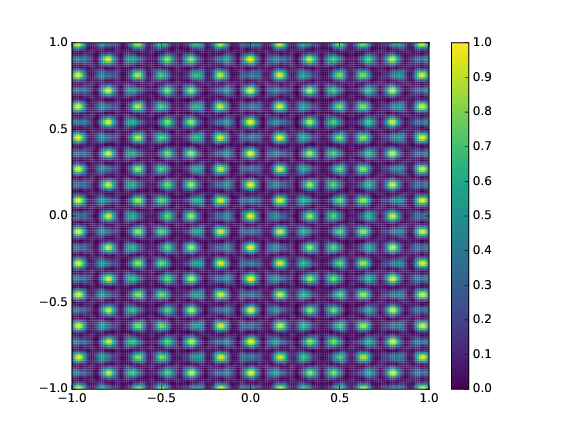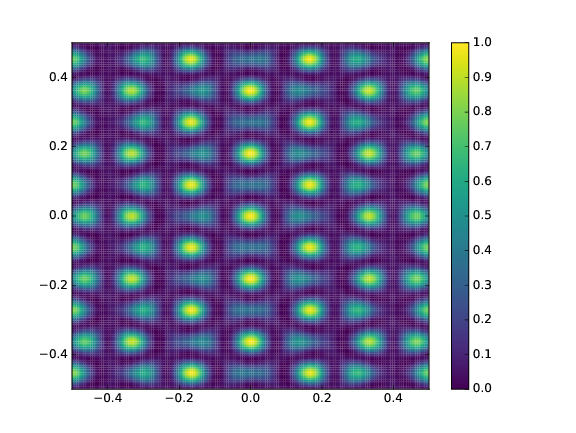# Array response

Hello members of Obspy,

I am starting to studying seismic arrays, so I decided to implement an array response of my deployment of ocean botton seismometers.

I attach a map and the script I ve used to calculate the array response. So I would like to know if someone could check if it is everything all right.

Moreover I would like to know if you could give me a hand with the fully understanding of the capabilities of this array. Because, from the array response I don’t fully unsestand if this array will be able to detect earthquakes (local/regional or telesismics) , what kind of waves I will be able to manage implementing a F-K analysis or vespagram.

Another question is, this array reponse is just for k(vector from the north) how can I know how well would be my array for differents backazimuth N—>E.

Thank you so much for any help

SCRIPT

import numpy as np
import matplotlib.pyplot as plt

from obspy.imaging.cm import obspy_sequential
from obspy.signal.array_analysis import array_transff_wavenumber

# generate array coordinates

coords = np.array([[-10.373608,35.909685, 0.021], [-10.554655,35.594688, 0.154], [-10.988262,35.595025,0.023],[-10.988500, 36.220166,0.204], [-10.555266,36.220216,0.003]])

#coords /= 1000.
klim = 0.5
kxmin = -klim
kxmax = klim
kymin = -klim
kymax = klim
kstep = klim / 100.
transff = array_transff_wavenumber(coords, klim, kstep, coordsys=‘lonlat’)

# plot

plt.pcolor(np.arange(kxmin, kxmax + kstep * 1.1, kstep) - kstep / 2.,
np.arange(kymin, kymax + kstep * 1.1, kstep) - kstep / 2.,
transff.T, cmap=obspy_sequential)
plt.colorbar()
plt.clim(vmin=0., vmax=1.)
plt.xlim(kxmin, kxmax)
plt.ylim(kymin, kymax)
plt.show()arrayresponse.py (838 Bytes)

Hi Roberto,

What you are plotting is a wavenumber transfer function, which is not very intuitive given that the wavenumber is the product of slowness and angular frequency.
Hence you have all frequency and slowness combinations bunched up.

What you are looking for is the slowness representation, which can be derived with the following function array_transff_freqslowness() in obspy.
This will give you an array response function in a certain frequency range, and show you the resolution capabilities for the ideal case.

Moreover I would like to know if you could give me a hand with the fully understanding of the capabilities of this array. Because, from the array response I don’t fully unsestand if this array will be able to detect earthquakes (local/regional or telesismics) , what kind of waves I will be able to manage implementing a F-K analysis or vespagram.

Given that the ocean bottom is a very noisy place and you have entered only 5 coordinates, which I assume your array is made of, you can expect a very poor resolution compared to land based arrays with more stations.
The performance can vary dependent on the location of the array (is it placed in a noisy environment?), the frequency of interest, amount of station, their respective spatial distribution, etc.
You can model the performance of your array under ideal conditions, but if the array can reach the ideal resolution under real conditions depends on the above factors.

Another question is, this array reponse is just for k(vector from the north) how can I know how well would be my array for differents backazimuth N—>E.

To my understanding, the ARF as calculated in your used obspy function is omnidirectional, but its interpretation of the k space is not intuitive.
Hence try the slowness representationCheers,
Martin